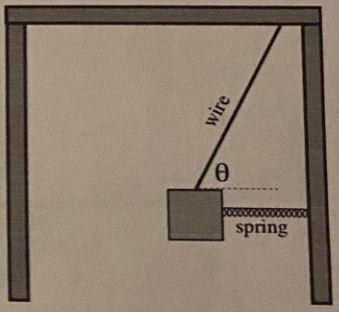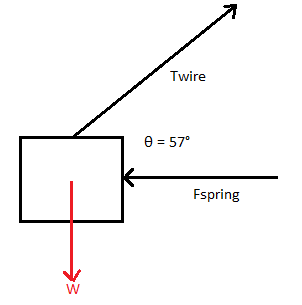# A block of mass 7.5 kg hangs from a wire as shown in the figure. A spring of stiffness 3500 N/m...

## Question:

A block of mass 7.5 kg hangs from a wire as shown in the figure. A spring of stiffness 3500 N/m pushes it away from the wall. The angle {eq}\theta {/eq} is 57 degrees.(a) Draw a free-body diagram showing all the forces on the block.

(b) What is the magnitude of the force exerted by the spring on the block?

(c) By how much is the (rather stiff) spring compressed?

(d) What is the tension in the wire?

## Hooke's Law:

Hooke's law which is named after the English scientist and architect Robert Hooke is a mathematical description of the relation between spring deformation to the force exerted by the spring. He discovered that the length of deformation is linear or directly proportional to the force of the spring.

Become a Study.com member to unlock this answer! Create your account

(a)(b)

Since the system is in equilibrium, we can apply Newton's second law of motion which states that the sum of all the forces in the...Hooke's Law & the Spring Constant: Definition & Equation

from

Chapter 4 / Lesson 19
202K

After watching this video, you will be able to explain what Hooke's Law is and use the equation for Hooke's Law to solve problems. A short quiz will follow.